# Level-set Geometry Description for the Finite Cell Method

Mohamed Elhaddad did his honours’ project at the Chair for Computation in Engineering (TUM) and
was supervised by Dipl. -Ing.Tino Bog, Nils Zander M.Sc. and Prof. Dr. rer. nat. Ernst Rank.

#### Introduction

The Finite element method has become the standard tool for obtaining approximate solutions to boundary value problems in computational mechanics. Problems with complex geometries require most of the effort for mesh generation. The finite cell method (FCM) avoids this challenge on the mesh level by embedding the geometry in a domain of simple shape . Geometry description implicitly using closed form expressions (only simple geometries), or voxel models. Alternatively, the level-set method can be used for an implicit geometry description. The use of a level-set geometry description within FCM would allow to easily handle problems where the boundary, or even the topology, changes during the simulation. In this honour’s project the level-set method is used in conjunction with FCM for non-linear free-surface seepage analysis.

#### Numerical Examples

Level-set functions define interfaces as the zero-level of a scalar function, where negative values are outside the domain and positive values lie inside. In the context of FCM, level-set functions could be easily used to define the geometry of the physical domain. Simply evaluating the level-function at a point, and checking the sign determines whether a point lies inside or outside the domain described by the level-set function.

FCM is employed to solve the problem of free-surface seepage through a dam.The governing differential equations define the interface (free-surface of water) as an explicit function of the pressure. This definition is used to define the level-set function, which is used to define the geometry . Applying FCM for spatial discretization leads to a system of non-linear equations, which is solved using a fix-point iteration scheme.

##### Rectangular Dam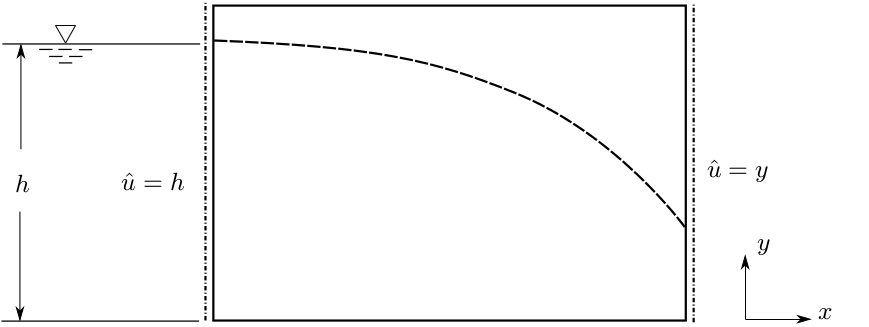Fig.1: Rectangular dam setup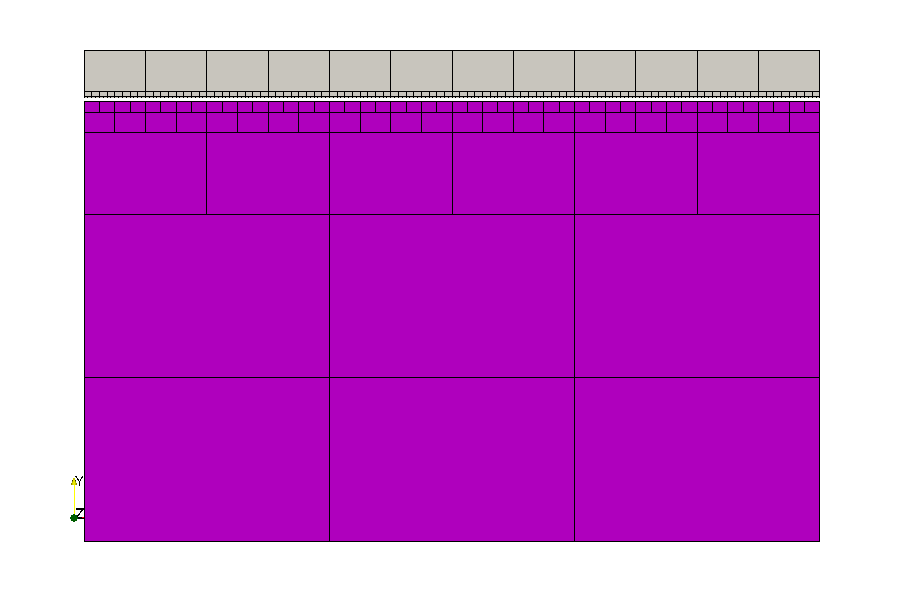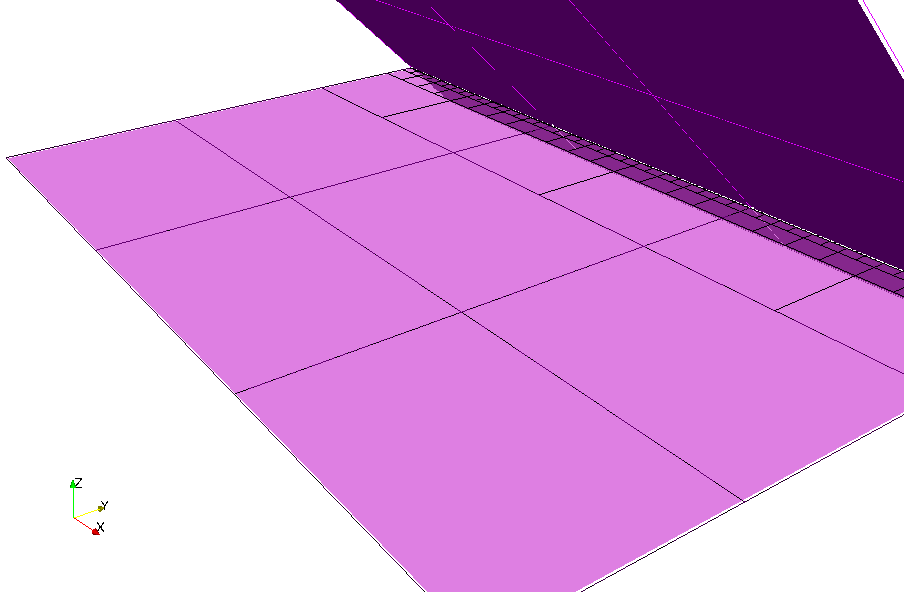(a) Pressure contours (b) Level-set

Fig.2: Solution with FCM

Difficulties in convergence were experienced, due to high oscillations of the solution in the fictitious domain, leading to “islands” in the fictitious domain with a positive level-set value, falsely identified as belonging to the physical domain.
Remedy: filtering technique to identify these “islands”: sampling several points on a line between each point with a positive level-set value and a predefined point on the bottom boundary to check if it is connected to the phyiscal domain. However, this filtering technique is numerically expensive, and not applicable for general cases.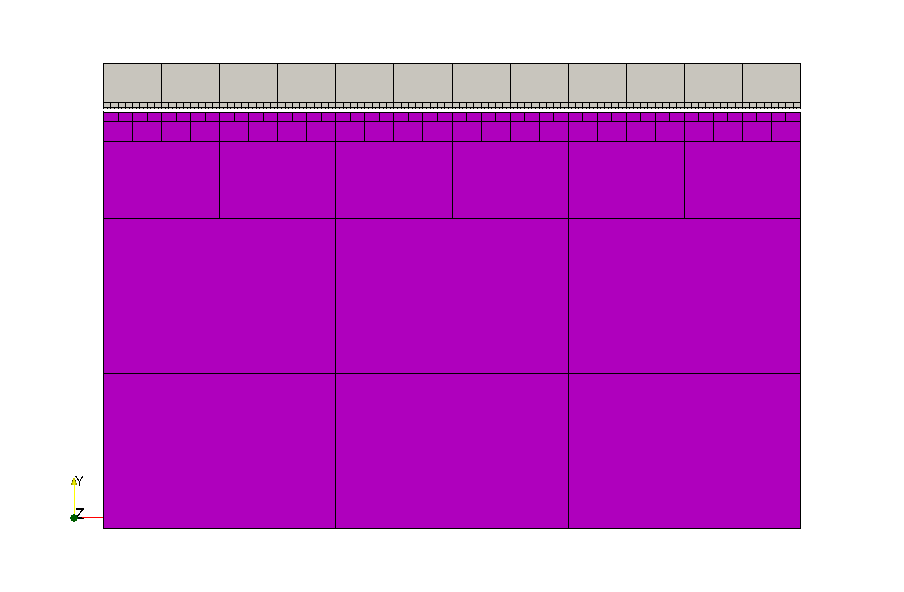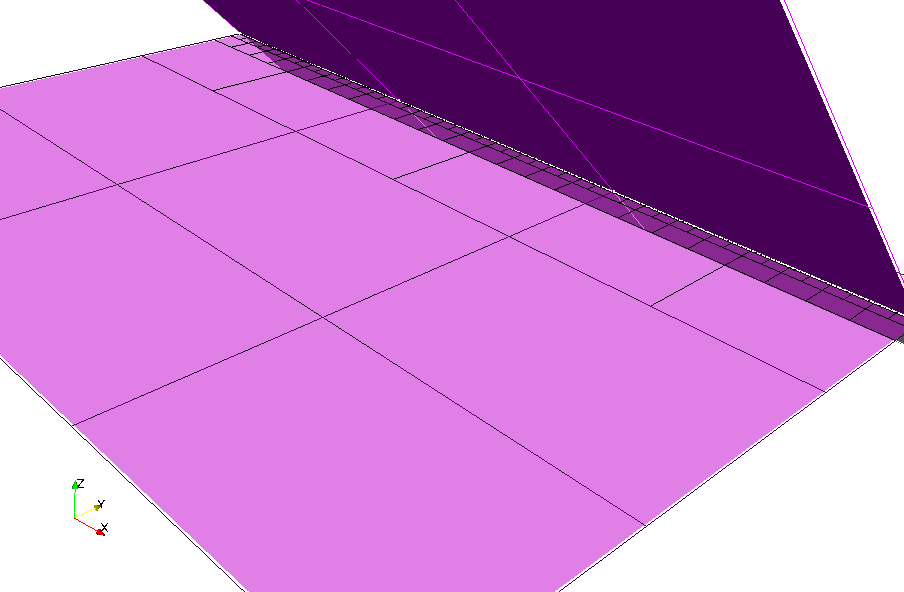(a) Pressure contours (b) Level-set

Fig.3: Solution with FCM using filtering technique

##### Analytical Benchmark

A benchmark problem is solved using FCM with a level-set geometry description, employing the filtering technique. A convergence study was carried outfor p- and h-refinement (Fig 4). The study shows that the method converges with the expected rates when the influence of the fictitous domain is properly controlled.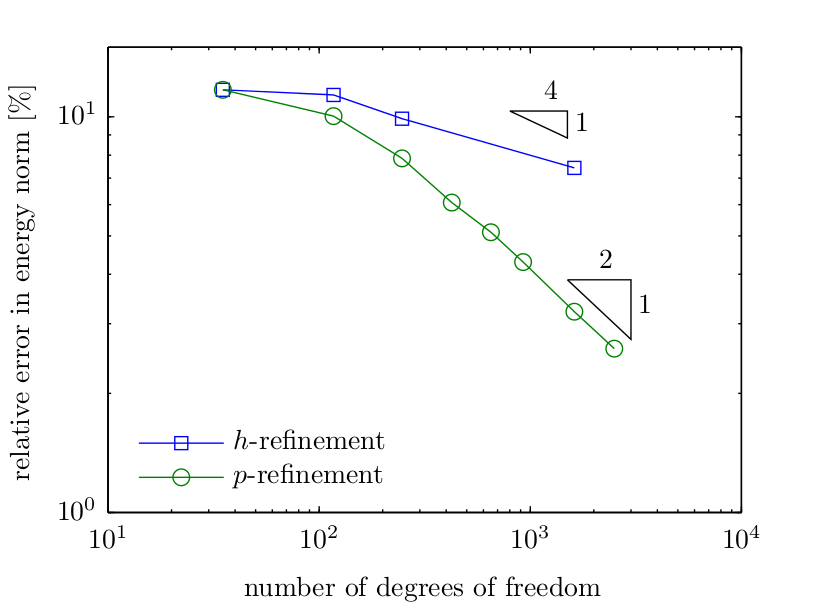Fig.4: Convergence in the energy norm

##### Trapezoidal Dam

The problem is solved for a trapezoidal dam embedded in a Cartesian mesh. The essential boundary conditions are enforced weakly using the penaalty method.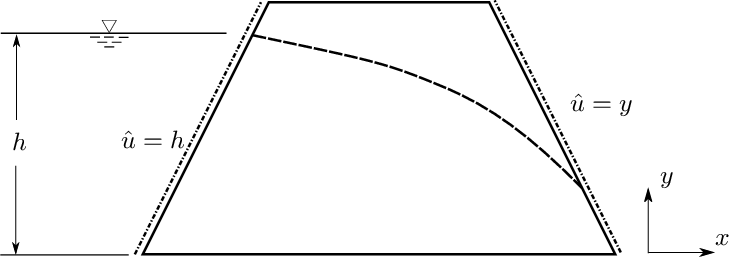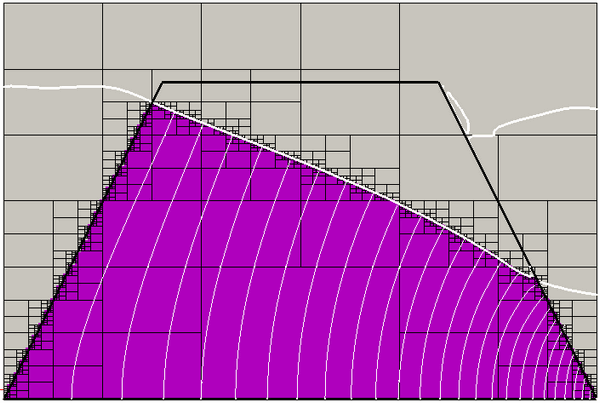(a) Analysis setup (b) Converged solution

Fig.5: Solution with FCM using filtering technique

#### Conclusions

A level-set description for geometry for the finite cell method was used to solve the free-surface seepage problem. Difficulties arise when values for the solution in the fictitious domain are used to determine the level-set function. To effectively use level-sets as a geometry-description within the FCM, the possible influence of solution values in the fictitious domain on the update of the level-set functions has to be adequately controlled.

#### References

  Rank, E.; Werner, H. An adaptive finite element approach for the free surface seepage problem. Int. J. for Numerical Methods in Eng., 23, 1986.  Parvizian, J.: Düster A.; Rank, E. Finite cell method. Computational Mechanics, 41(1): 121-133, December 2007. [ DOI | http ]